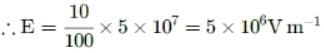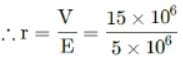In a Van de Graaff type generator, a spherical metal shell is to be 15 × 106 V electrode. The dielectric strength of the gas surrounding the electrode is 5 × 107 Vm−1. The minimum radius of the spherical shell required is
• a)
1 m
• b)
2 m
• c)
1.5 m
• d)
3 m

### Related TestVp Classes Jan 20, 2022Here, V = 15 × 106 V, dielectric strength
= 5 x 107 V m-1
Maximum electric field, E = 10% of dielectric strengthAs E = V/r= 3 m

Here, V = 15 × 106 V, dielectric strength= 5 x 107 V m-1Maximum electric field, E = 10% of dielectric strengthAs E = V/r= 3 m
Do you know? How Toppers prepare for NEET Exam
With help of the best NEET teachers & toppers, We have prepared a guide for student who are preparing for NEET : 15 Steps to clear NEET Exam